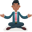Trusted answers to developer questions
Trusted Answers to Developer Questions

Related Tags

redis

# What are sorted sets in Redis?Abhilash

Grokking Modern System Design Interview for Engineers & Managers

Ace your System Design Interview and take your career to the next level. Learn to handle the design of applications like Netflix, Quora, Facebook, Uber, and many more in a 45-min interview. Learn the RESHADED framework for architecting web-scale applications by determining requirements, constraints, and assumptions before diving into a step-by-step design process.

### Sorted sets in Redis

Let’s break the word “sorted set” into two words, sorted, which means order, and set, which means the items are unique. Hence, a sorted set is a data structure in Redis that stores unique values in a sorted way.

Every member in the sorted set is associated with a score that determines its order among other elements in the set.

Some of the essential commands associated with sorted sets are as follows:

### The ZADD command

The ZADD command creates and adds one or member to the sorted set.

The syntax is as follows:

ZADD key score member


Where:

• key: This parameter is the name under which the sorted set is registered.
• score: This parameter is the score value associated with the member.
• member: This parameter is the member to add.

### The ZSCORE command

The ZSCORE command retrieves the score associated with the given member.

The syntax is as follows:

ZSCORE key member


Where:

• key: This parameter is the name under which the sorted set is registered.
• member: This parameter is the member for which the score has to be retrieved.

### The ZRANK command

The ZRANK command is used to retrieve the index of the member in the sorted set ordered from low to index. The rank or the index is zero-based, i.e., the rank of the member with the lowest score is 0.

The syntax is as follows:

ZRANK key member


Where:

• key: This parameter is the name under which the sorted set is registered.
• member: This parameter is the member for which the rank has to be retrieved.

### The ZREM command

The ZREM command removes the given member from the sorted set.

The syntax is as follows:

ZREM key member


Where:

• key: This parameter is the name under which the sorted set is registered.
• member: This parameter is the member to be removed.

### The ZRANGE command

The ZRANGE command is used to retrieve all the members of the sorted set that fall in the range of the given values.

The syntax is as follows:

ZRANGE key min max


Where:

• key: This parameter is the name under which the sorted set is registered.
• min: This parameter is the minimum value.
• max: This parameter is the maximum value.

### The ZINCRBY command

The ZINCRBY command is used to increment the member’s score by the specified amount.

The syntax is as follows:

ZINCRBY key increment member


Where:

• key: This parameter is the name under which the sorted set is registered.
• increment: This parameter is the amount to increment.
• member: This parameter is the sorted set member.

### The ZCOUNT command

The ZCOUNT command retrieves the count of members with a score between min and max.

The syntax is as follows:

ZCOUNT key min max


Where:

• key: This parameter is the name under which the sorted set is registered.
• min: This parameter is the minimum value.
• max: This parameter is the maximum value.

### The ZCARD command

The ZCARD command retrieves the number of members in the sorted set.

The syntax is as follows:

ZCARD key


Where:

• key: This parameter is the name under which the sorted set is registered.

Run the above commands in the following terminal.

Terminal 1
Terminal

RELATED TAGS

redis

CONTRIBUTORAbhilash